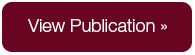# Papers

## Ruling Out Multiplicity of Smooth Equilibria in Dynamic Games: A Hyperbolic Discounting Example”

Serguei Maliar and Lilia MaliarAbstract

The literature that conducts numerical analysis of equilibrium in models with hyperbolic (quasi-geometric) discounting reports difficulties in achieving convergence. Surprisingly, numerical methods fail to converge even in a simple, deterministic optimal growth problem that has a well-behaved, smooth closed-form solution. We argue that the reason for nonconvergence is that the generalized Euler equation has a continuum of smooth solutions, each of which is characterized by a different integration constant. We propose two types of restrictions that can rule out the multiplicity: boundary conditions and shape restrictions on equilibrium policy functions. With these additional restrictions, the studied numerical methods deliver a unique smooth solution for both the deterministic and stochastic problems in a wide range of the model’s parameters.